GRE Subject Test: Math : Limits of Sequences

Example Questions

Example Question #26 : Series In Calculus

There are 2 series,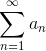and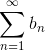, and they are both convergent. Is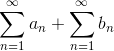convergent, divergent, or inconclusive?

Divergent

Inconclusive

Convergent

Convergent

Explanation:

Infinite series can be added and subtracted with each other.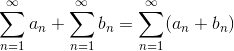Since the 2 series are convergent, the sum of the convergent infinite series is also convergent.

Note: The starting value, in this case n=1, must be the same before adding infinite series together.

Example Question #27 : Series In Calculus

You have a divergent series, and you multiply it by a constant 10. Is the new series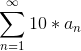convergent or divergent?

Convergent

Divergent

Inconclusive

Divergent

Explanation:

This is a fundamental property of series.

For any constant c, ifis convergent then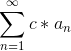is convergent, and ifis divergent,is divergent.is divergent in the question, and the constant c is 10 in this case, sois also divergent.

Example Question #161 : Gre Subject Test: Math

There are 2 series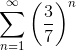and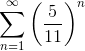.

Is the sum of these 2 infinite series convergent, divergent, or inconclusive?

Divergent

Convergent

Inconclusive

Convergent

Explanation:

A way to find out if the sum of the 2 infinite series is convergent or not is to find out whether the individual infinite series are convergent or not.

Test the first series.

This is a geometric series with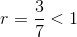.

By the geometric test, this series is convergent.

Test the second series.

This is a geometric series with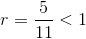.

By the geometric test, this series is convergent.

Since both of the series are convergent,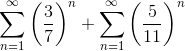is also convergent.

Example Question #1 : Sequences & Series

Find the radius of convergence for the power series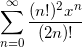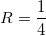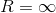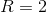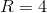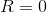Explanation:

We can use the limit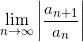to find the radius of convergence. We have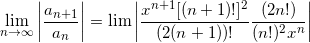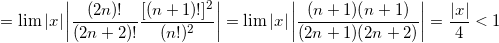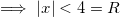This means the radius of convergence is.

Example Question #67 : Convergence And Divergence

Determine if the following series is divergent, convergent or neither.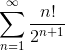Divergent

Neither

Both

Inconclusive

Convergent

Divergent

Explanation:

In order to figure ifis convergent, divergent or neither, we need to use the ratio test.

Remember that the ratio test is as follows.

Suppose we have a series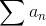. We define,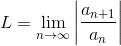Then if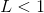, the series is absolutely convergent.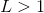, the series is divergent.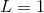, the series may be divergent, conditionally convergent, or absolutely convergent.

Now lets apply the ratio test to our problem.

Let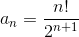and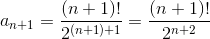Now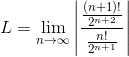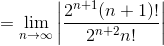.

Now lets simplify this expression to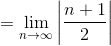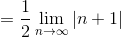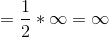.

Since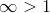,

we have sufficient evidence to conclude that the series is divergent.

Example Question #2 : Sequences & Series

Calculate the sum of the following infinite geometric series: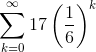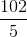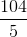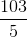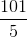Explanation:

This is an infinite geometric series.

The sum of an infinite geometric series can be calculated with the following formula,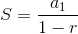, where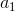is the first value of the summation, and r is the common ratio.

Solution:

Value ofcan be found by setting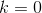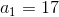r is the value contained in the exponent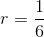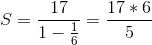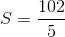Example Question #62 : Types Of Series

Determine how many terms need to be added to approximate the following series within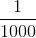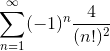Explanation:

This is an alternating series test.

In order to find the terms necessary to approximate the series withinfirst see if the series is convergent using the alternating series test. If the series converges, find n such that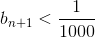Step 1:

An alternating series can be identified because terms in the series will “alternate” between + and –, because of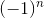Note: Alternating Series Test can only show convergence. It cannot show divergence.

If the following 2 tests are true, the alternating series converges.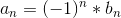1.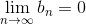2.        {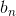} is a decreasing sequence, or in other words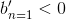Solution: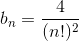1.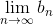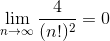2. {} is a decreasing functon, since a factorial never decreases.

Since the 2 tests pass, this series is convergent.

Step 2:

Plug in n values until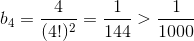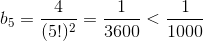4 needs to be added to approximate the sum within.

Example Question #1 : Sequences & Series

Evaluate: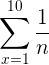. (Round to 4 places)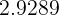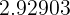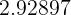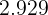Explanation:

Step 1: Plug in values into the function and add up the fraction: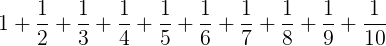Step 2: Find the sum of the fractions....

We can convert the fractions to decimals: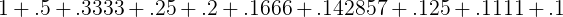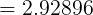Step 3: Round to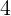places...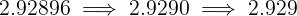All GRE Subject Test: Math Resources